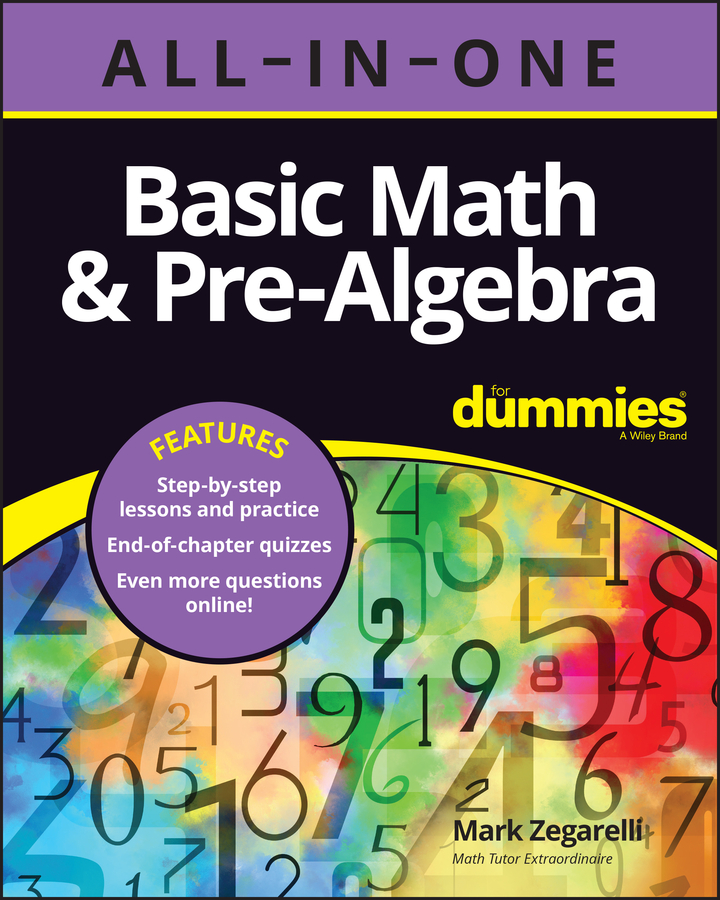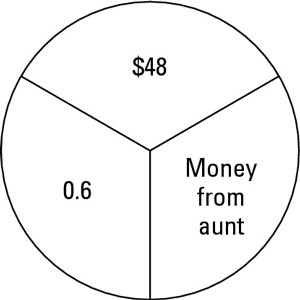##### Basic Math & Pre-Algebra All-in-One For Dummies (+ Chapter Quizzes Online)Some math word problems give you the amount that you end up with and ask you to find out how much you started with. These problems can be difficult if you’re not used to thinking backwards. Here’s an example, and it’s kind of a tough one, so fasten your seat belt:

Maria received some birthday money from her aunt. She put 40% in the bank, spent 75% of the rest on a purse, and when she was done, she had \$12 left to spend on dinner. How much did her aunt give her?

In this problem, you need to start at the end and work backwards. Notice that the only dollar amount in the problem comes after the two percent amounts. The problem tells you that she ends up with \$12 after two transactions — putting money in the bank and buying a purse — and asks you to find out how much she started with.

To solve this problem, set up two word equations to describe the two transactions:

Money from aunt – money for bank = money after bank

Money after bank – money for purse = \$12

Notice what these two word equations are saying. The first tells you that Maria took the money from her aunt, subtracted some money to put in the bank, and left the bank with a new amount of money, which is called money after bank. The second word equation starts where the first leaves off. It tells you that Maria took the money left over from the bank, subtracted some money for a purse, and ended up with \$12.

This second equation already has an amount of money filled in, so start here. To solve this problem, realize that Maria spent 75% of her money at that time on the purse — that is, 75% of the money she still had after the bank:

Money after bank – 75% of money after bank = \$12

This example is going to make one small change to this equation so you can see what it’s really saying:

100% of money after bank – 75% of money after bank = \$12

Adding 100% of doesn’t change the equation because it really just means you’re multiplying by 1. In fact, you can slip these two words in anywhere without changing what you mean, though you may sound ridiculous saying “Last night, I drove 100% of my car home from work, walked 100% of my dog, then took 100% of my wife to see 100% of a movie.”

In this particular case, however, these words help you to make a connection because 100% – 75% = 25%. Here’s an even better way to write this equation:

25% money after bank = \$12

This tells you that \$12 divided by 0.25 gives you the remaining amount of \$48.

Okay, you know how much money Maria had after making the bank deposit, so you can plug this number into the first equation:

Money from aunt – money for bank = \$48

Now you can use the same type of thinking to solve this equation (and it goes a lot more quickly this time!). First, Maria placed 40% of the money from her aunt in the bank:

Money from aunt – 40% of money from aunt = \$48

Again, rewrite this equation to make what it’s saying clearer:

100% of money from aunt – 40% of money from aunt = \$48

Now, because 100% – 40% = 60%, rewrite it again:

60% money from aunt = \$48Money from aunt = \$48 ÷ 0.6 = \$80.
At this point, you can use the above percent circle to solve the equation. In this case, the percent circle tells you that \$48 divided by 0.6 gives you the remaining amount of \$80. So Maria’s aunt gave her \$80 for her birthday.